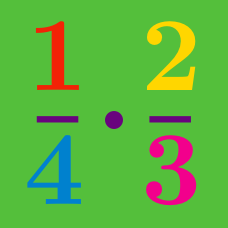Basic Mathematics

# Fractions: Level 2 ChallengesA waiter's income consists of his salary and tips made. During one week his tips were 5/4 of his salary . What fraction of his income came from tips?

If $\color{#D61F06}{a}$ and $\color{#3D99F6}{b}$ are positive, what is the minimum possible value of $\large \frac { \color{#D61F06}{a}}{ \color{#3D99F6}{b} } +\frac { \color{#3D99F6}{b} }{\color{#D61F06}{a} }?$

Paul try to solve the value of $\frac{x+y}{z}$ using a calculator.

He inputs $x+y \div z$ and the result is $12$.

For clarification, he inputs $y+x \div z$ and the result is $9$.

Lastly, Paul concludes that his input is wrong.

So, he input $(x+y) \div z$ and the result is $7$.

In the story, find the value of $x+y+z$.

$x=\dfrac{111110}{111111} , y = \dfrac{222221}{222223} , z = \dfrac{333331}{333334}$

Compare $x,y,z$.

Find the positive integer $\color{#3D99F6}{X}$ that makes this equation true:

$\Large \frac { 2 }{ \color{#3D99F6}{X} } -\frac { \color{#3D99F6}{X}}{ 5 } =\frac { 1 }{ 15 }.$

×

Problem Loading...

Note Loading...

Set Loading...